# What is EDA

In this class we’ll focus on the Exploratory Data Analysis (EDA). In short, it refers to what we have been doing so far: data transformation and data visualization. We are going to dive deeper into those two but in a more coherent framework so that you become as comfortable as possible with data exploration in R. Nothing fundamentally new in this lab – we’ll only dive deeper into using the tools we already know.

You should think of the goal of exploratory data analysis as the following: you want to explore as many aspects as possible in your data so that you gain a better understanding of what is already there. The way you go about this is by so many iterations of questions -> visualizations to answer those questions.

# ggplo2: one more time

First, let’s revisit some basics of ggplot2 library. We’ll use a new dataset called `mpg`. The dataset comes from the US Enviromental Protection Agency which collected a couple of models along with the following features for each model: manufacturer, model, year, engine size in liters, transmission type along with other variables.

``library(ggplot2)``

You can read more about the dataset by typing this command in the console:

``?mpg``

Let’s look at the first few lines:

``mpg``
``````## # A tibble: 234 x 11
##    manufacturer model displ  year   cyl trans drv     cty   hwy fl    class
##    <chr>        <chr> <dbl> <int> <int> <chr> <chr> <int> <int> <chr> <chr>
##  1 audi         a4      1.8  1999     4 auto… f        18    29 p     comp…
##  2 audi         a4      1.8  1999     4 manu… f        21    29 p     comp…
##  3 audi         a4      2    2008     4 manu… f        20    31 p     comp…
##  4 audi         a4      2    2008     4 auto… f        21    30 p     comp…
##  5 audi         a4      2.8  1999     6 auto… f        16    26 p     comp…
##  6 audi         a4      2.8  1999     6 manu… f        18    26 p     comp…
##  7 audi         a4      3.1  2008     6 auto… f        18    27 p     comp…
##  8 audi         a4 q…   1.8  1999     4 manu… 4        18    26 p     comp…
##  9 audi         a4 q…   1.8  1999     4 auto… 4        16    25 p     comp…
## 10 audi         a4 q…   2    2008     4 manu… 4        20    28 p     comp…
## # … with 224 more rows``````

We can asl a very simple question about fuel efficiency: what is the relationship between car engine size (i.e., `displ`) and fuel consumption (i.e., `hwy`)? We can simply answer this question using the two variables: displ (which codes for engine size in liters) and hwy (which codes for fuel efficiancy in miles per gallon in highways). As both variables are continous numbers, we can use a scatter plot:

``````ggplot(mpg, aes(x=displ, y=hwy)) +
geom_point()``````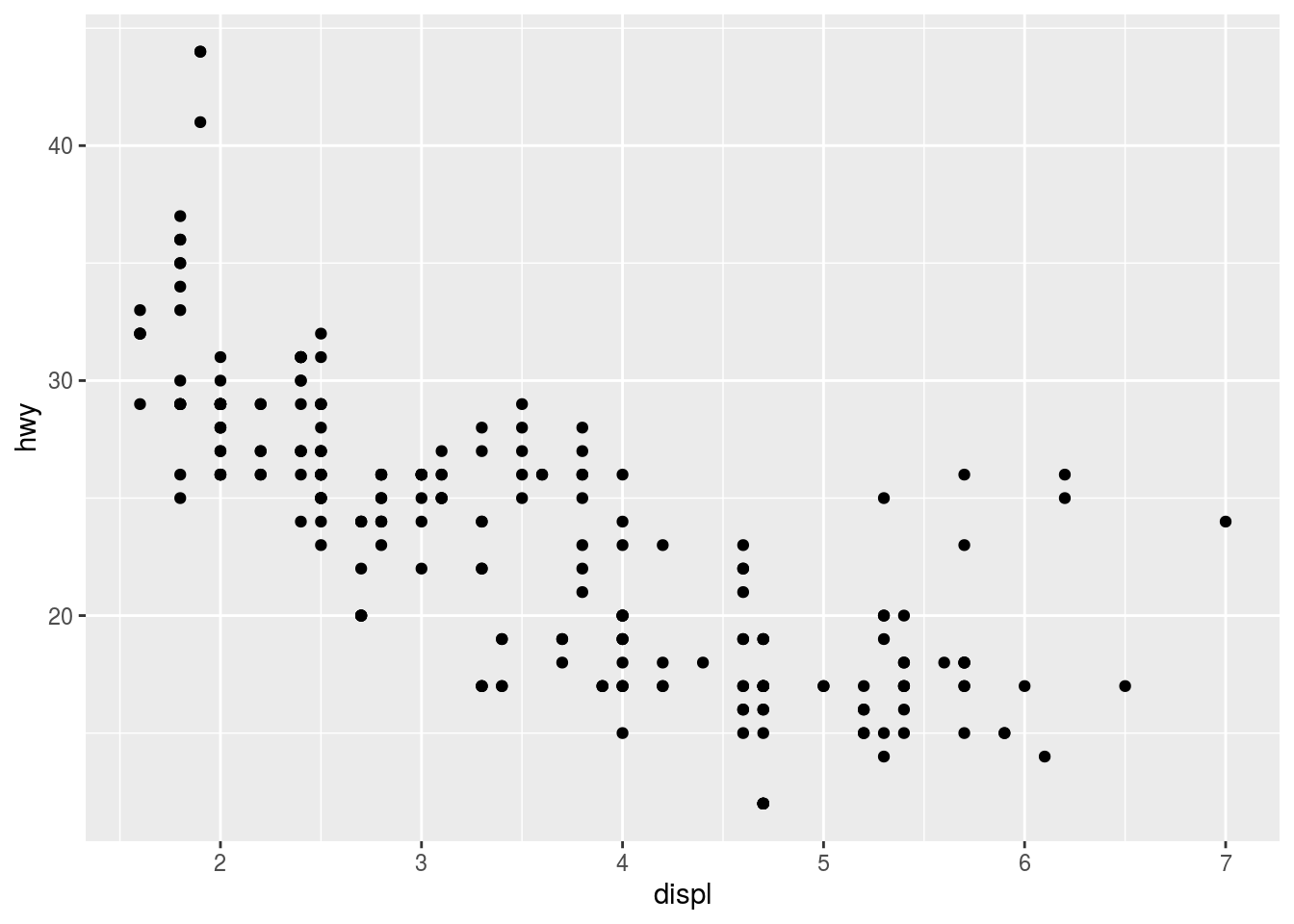As you see, we see a negative relationship between engine size and fuel efficincy. Now we can cliam that we are done with the first iteration of Q->V routine. We’ll do that throughout this lesson.

## plotting 3 variables

In ggplot2, we have a lot more options we’ll explore in this short tutorial. Let’s vary the points in the scatter plots by a third variable. For example, we can color those cars using the class of the car:

``````ggplot(mpg, aes(x=displ, y=hwy, color=class)) +
geom_point()``````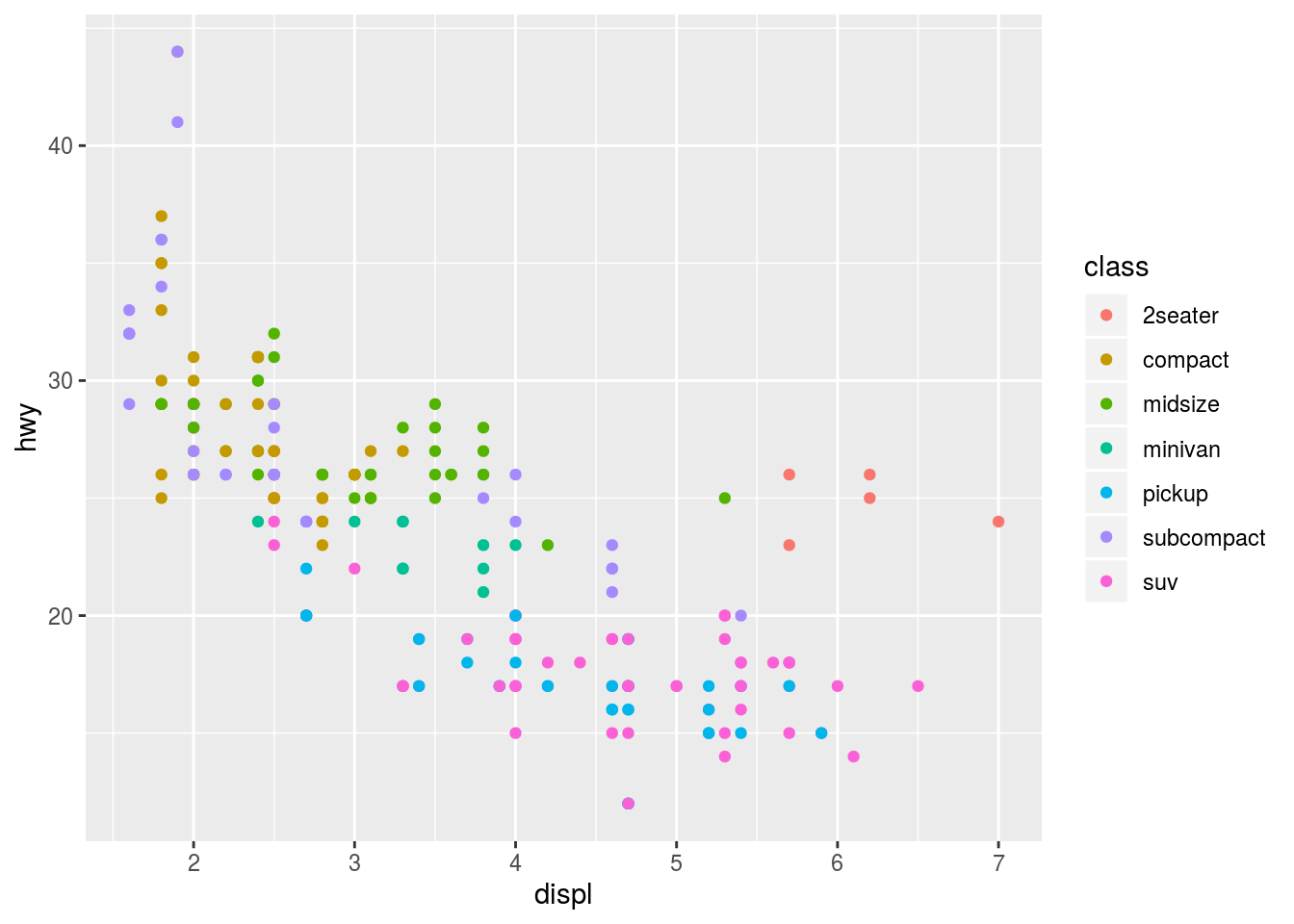All what we did was adding `color=class` inside the `aes` command in the plotting command. We can also use `shape` as well

``````ggplot(mpg, aes(x=displ, y=hwy, shape=class)) +
geom_point()``````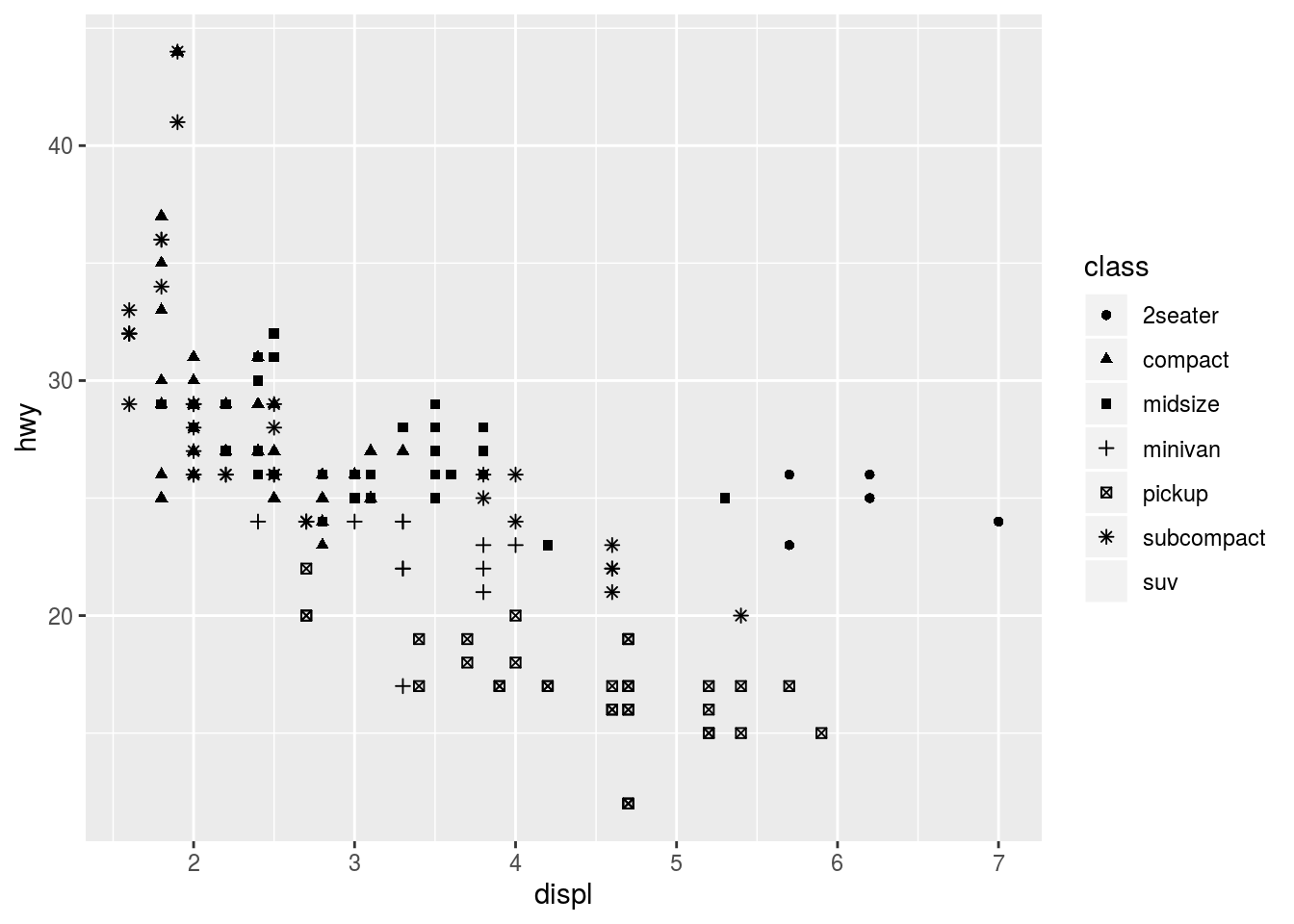If the third variable was continous (made of numbers), then we can use other options including size (which does not make much sense if your third variable is categorical):

``````ggplot(mpg, aes(x=displ, y=hwy, size=class)) +
geom_point()``````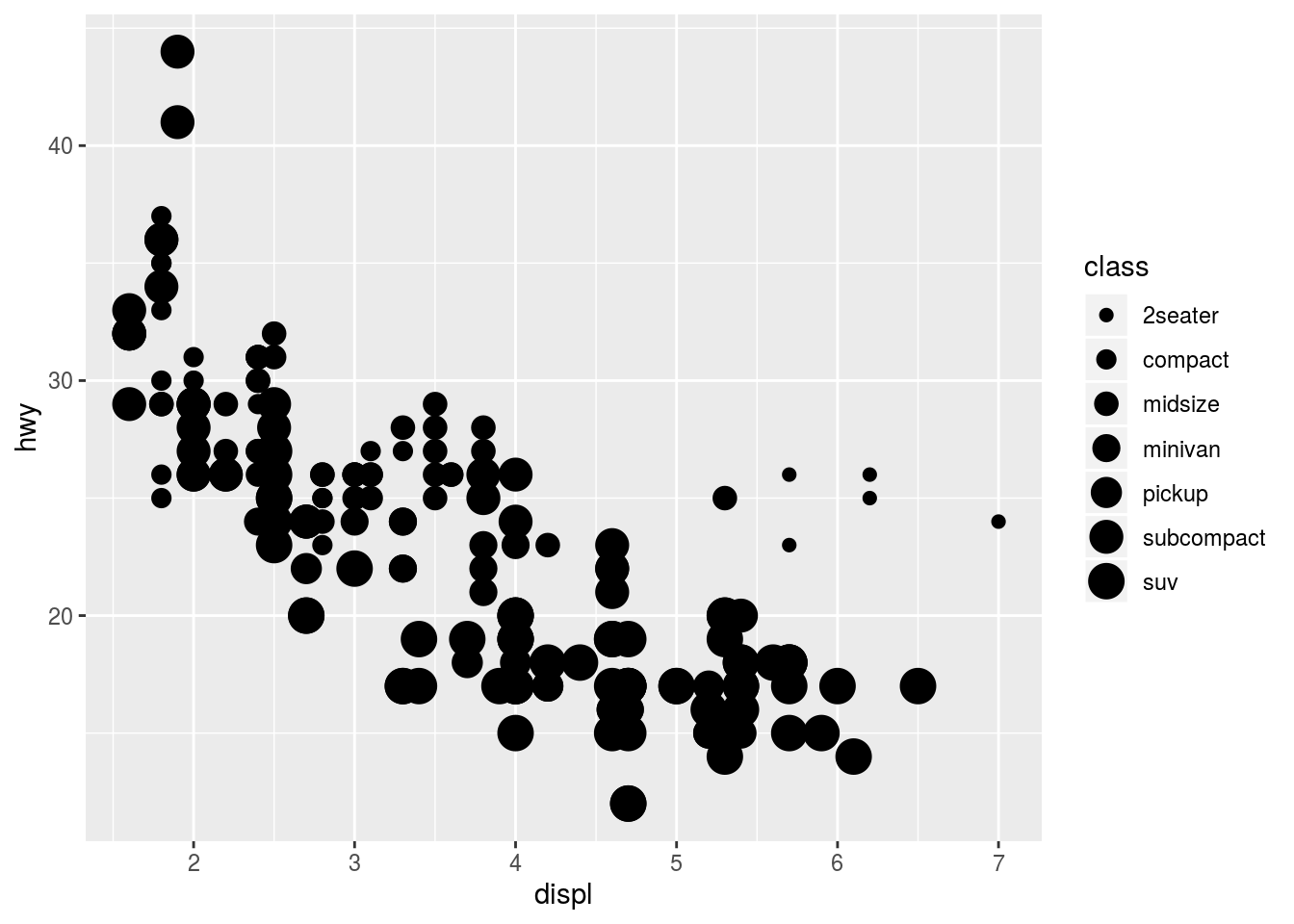or alpha:

``````ggplot(mpg, aes(x=displ, y=hwy, alpha=class)) +
geom_point()``````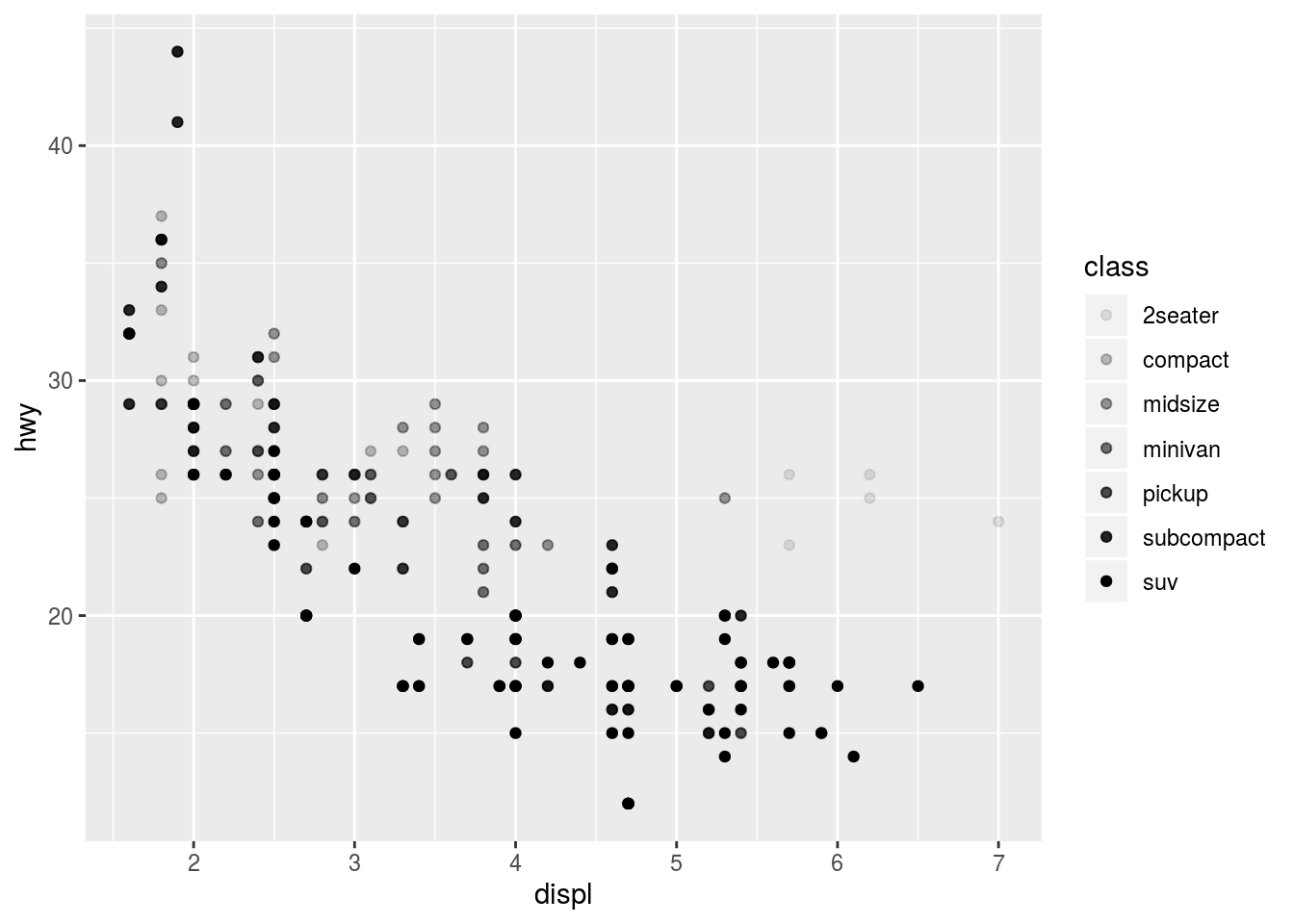## faceting

Another feature of ggplot is called faceting. It is the splitting of a plot into many subplots by a given thrid variable. For example, we can plot the same relationship but by making a small subplot for each class using the function `facet_wrap`:

``````ggplot(mpg, aes(x=displ, y=hwy, color=class)) +
geom_point() +
facet_wrap(~class, nrow=2)``````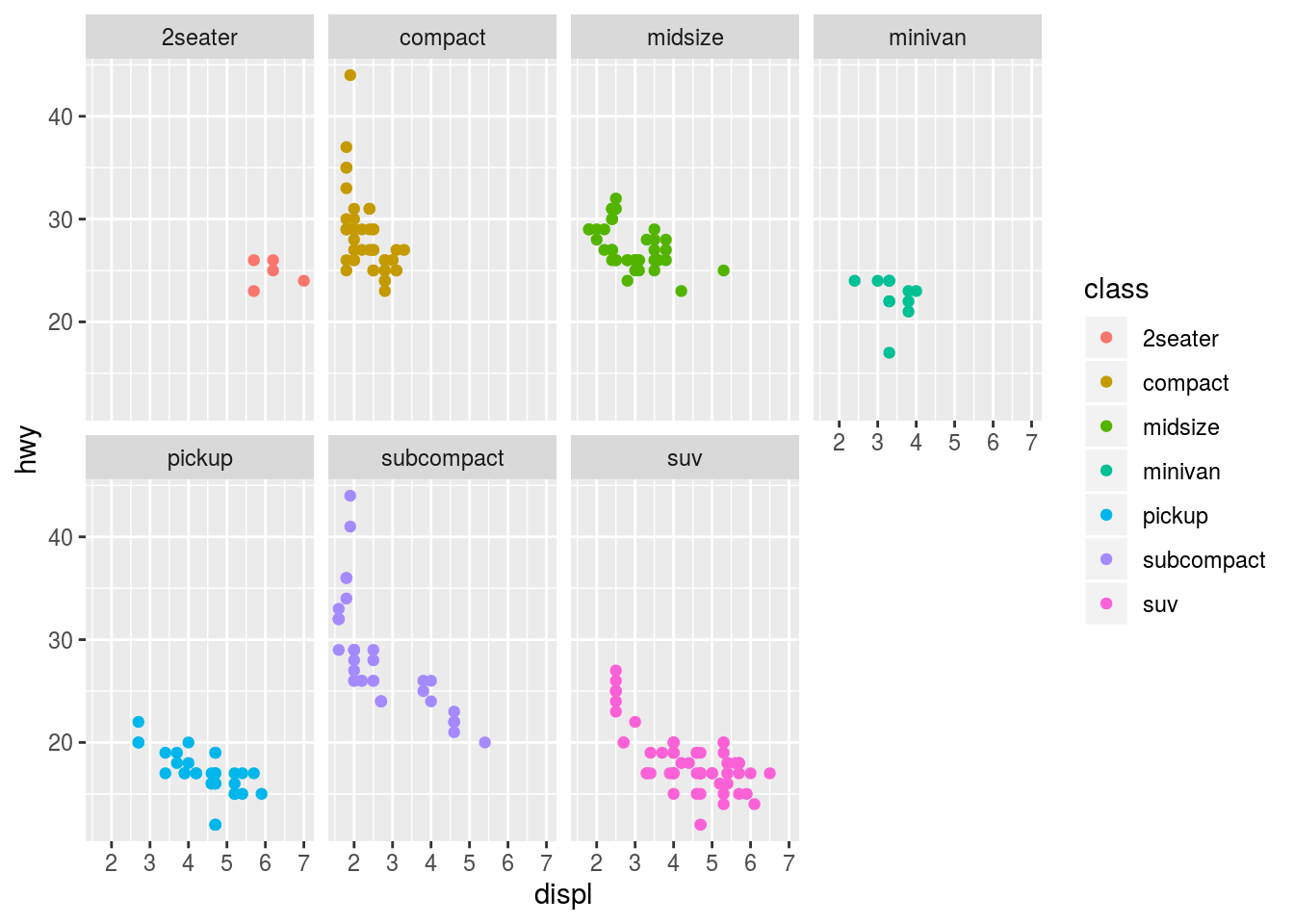And now we can see the relationship clearer. You can also use a combination of two variables by writing the varialbes around the telda ~ symbol

``````ggplot(mpg, aes(x=displ, y=hwy)) +
geom_point() +
facet_wrap(cyl~class)``````